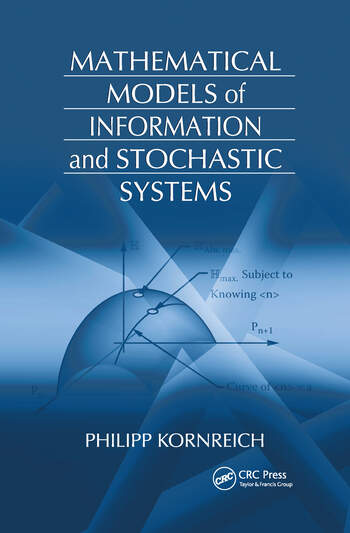# Mathematical Models of Information and Stochastic Systems

## 1st Edition

Philipp Kornreich

CRC Press
Published May 13, 2008
Textbook - 376 Pages - 124 B/W Illustrations
ISBN 9781420058833 - CAT# 58835

For Instructors Request Inspection Copy

was \$140.00

USD\$112.00

SAVE ~\$28.00

Currently out of stock
FREE Standard Shipping!

## Preview

### Summary

From ancient soothsayers and astrologists to today’s pollsters and economists, probability theory has long been used to predict the future on the basis of past and present knowledge. Mathematical Models of Information and Stochastic Systems shows that the amount of knowledge about a system plays an important role in the mathematical models used to foretell the future of the system. It explains how this known quantity of information is used to derive a system’s probabilistic properties.

After an introduction, the book presents several basic principles that are employed in the remainder of the text to develop useful examples of probability theory. It examines both discrete and continuous distribution functions and random variables, followed by a chapter on the average values, correlations, and covariances of functions of variables as well as the probabilistic mathematical model of quantum mechanics. The author then explores the concepts of randomness and entropy and derives various discrete probabilities and continuous probability density functions from what is known about a particular stochastic system. The final chapters discuss information of discrete and continuous systems, time-dependent stochastic processes, data analysis, and chaotic systems and fractals.

By building a range of probability distributions based on prior knowledge of the problem, this classroom-tested text illustrates how to predict the behavior of diverse systems. A solutions manual is available for qualifying instructors.

#### Instructors

We provide complimentary e-inspection copies of primary textbooks to instructors considering our books for course adoption.